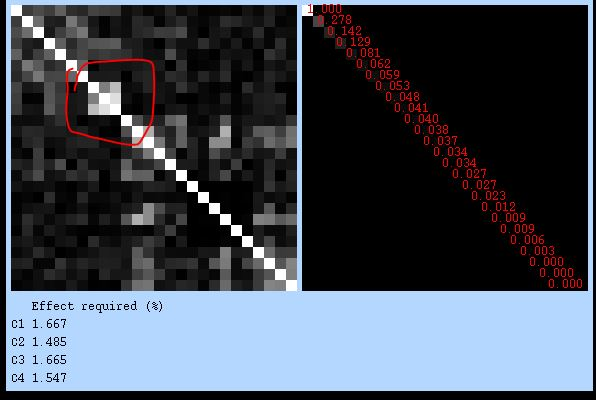# Effect of collinearity between error trials (nuisance) regressors on parameter estimates of regressors of interests

Dear fmri experts,

I am analyzing an event related task (Monetary Incentive Delay) with FSL’s FEAT and encountered an issue about collinearity between regressors of error trials which are introduced as part of the model regressors (i.e. not loaded through the confound EV list) but I am not intersted in them.
Each trial has three conditions - anticipation, target and consumption.
When subjects repond erroneously, I wish to regress out the whole trial. However, a previous analysis of this same task that I wish to adhere to (unless it’s incorrect), created a seperate error regressor for each type of condition - anticipation, target and consumption, resulting in 3 error regressors. I think this was out of coding convenience.
I did the same but when inspecting the covariance matrix of my level 1 models, I always see high collinearity between these three regreoors:This makes sense. The question is - what is the effect of collinearity between such nuisanse regressors on my parameter estimates and contrast maps, and on the variance maps (varCOPES)? Do I need to worry if repeatedly I see this pattern of covariance in almost all of my subjects? Will it influence my group level statistics (assuming I am using FLAME 1 mixed effects analysis on the group level, which uses both the COPEs and the varCOPEs to estimate the group variance)?